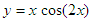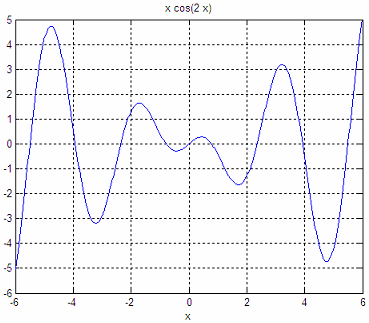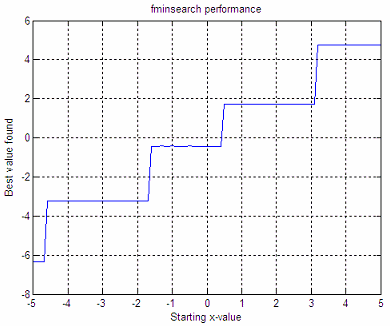# Mathematical Optimization

 Matlab includes at least two standard functions intended for numerical or mathematical optimization. These instructions are ‘fminbnd’ (for one single variable) and ‘fminsearch’ (for one or more variables). Built-in function ‘fminbnd’ tries to find a minimum of a function of one variable within a fixed interval.

Built-in function ‘fminsearch’ finds the minimum of a scalar function of several variables, starting at an initial estimate. This can be also considered as unconstrained nonlinear optimization. Both functions obtain a local minimum, not a global one.

Since we can explore and express a function in terms of a predefined error, minimizing this error function equals optimizing the function.

Let’s work with this expression:It can be defined in this way:

function y = xcos2(x)
y = x*cos(2*x);

and it can be easily plotted like this:

ezplot('x*cos(2*x)')
axis([-6 6 -6 5])
grid
onWe can implement a minimization method in the range [-5, 0], like this (the fminbnd algorithm is based on golden section search and parabolic interpolation):

options = optimset('Display','iter','TolX',0.001);
[xc, FunVal, EF, output] = fminbnd(‘xcos2’, -5, 0, options)

Matlab displays the following answer. We get the number of times that the function was evaluated, the point of evaluation and the function value at each iterate.

Func-count     x          f(x)         Procedure
1       -3.09017     -3.07384        initial
2       -1.90983      1.48735        golden
3       -3.81966    -0.813651        golden
4       -3.02998     -2.95481        parabolic
5       -3.21391     -3.18035        parabolic
6       -3.22291     -3.18038        parabolic
7       -3.21865      -3.1805        parabolic
8       -3.21832      -3.1805        parabolic
9       -3.21899      -3.1805        parabolic

Optimization terminated:
the current x satisfies the termination criteria using OPTIONS.TolX of 1.000000e-003

xc = -3.2187
FunVal = -3.1805
EF = 1
output =

iterations: 8
funcCount: 9
algorithm: 'golden section search, parabolic interpolation'
message: [1x112 char]

The value xc = -3.2187 is the best value found after our mathematical optimization. If we change the ‘TolX’ parameter of the options included in the optimization function (fminbnd), we get a different amount of function evaluations and, naturally, a slightly different minimum value. We must be aware that changes in tolerances naturally affect our results.

options = optimset('Display','iter','TolX',0.1);

Func-count     x          f(x)         Procedure
1       -3.09017     -3.07384        initial
2       -1.90983      1.48735        golden
3       -3.81966    -0.813651        golden
4       -3.02998     -2.95481        parabolic
5       -3.21391     -3.18035        parabolic
6       -3.24725     -3.17502        parabolic
7       -3.18058     -3.17092        parabolic

Optimization terminated:

the current x satisfies the termination criteria using OPTIONS.TolX of 1.000000e-001

xc = -3.2139
FunVal = -3.1804
EF = 1
output =
iterations: 6
funcCount: 7
algorithm: 'golden section search, parabolic interpolation'
message: [1x112 char]

If we now change the range of exploration to [0, 6], we find another minimum:

Func-count     x          f(x)         Procedure
1        3.81966     0.813651        initial
2        6.18034      6.05006        golden
3        2.36068    0.0211764        golden
4        2.47085     0.561649        parabolic
5        1.45898     -1.42265        golden
6        1.66467     -1.63542        parabolic
7        1.69841     -1.64339        parabolic
8        1.73174      -1.6428        parabolic

Optimization terminated:
the current x satisfies the termination criteria using OPTIONS.TolX of 1.000000e-001

xc = 1.6984
FunVal = -1.6434
EF = 1
output =
iterations: 7
funcCount: 8
algorithm: 'golden section search, parabolic interpolation'
message: [1x112 char]

Now, let’s explore the same function with fminsearch. We’re going to run different starting points, with this code:

fun = 'xcos2';
options = optimset(
'Display','iter','TolX',0.1);

i = 1;
for sx = -5 : .1 : 5
[xc, FunVal, EF, output] = fminsearch(fun, sx, options);
x(i) = sx;
xo(i) = xc;
oi(i) = output.iterations;
of(i) = output.funcCount;
i = i+1;

end

We get these graphics:These graphics say that if we start with x = -3, the minimum found after our mathematical optimization is y = -3.22, it takes 6 iterations to reach that value, and 12 function evaluations are needed to reach that minimum. If we start with x = 4, the minimum found is 4.8, it takes 9 iterations, and 18 function evaluations to reach that minimum.

We must be very aware that different initial conditions and tolerances lead us to different results. Mathematical optimization is not straigthforward most of the times.

From 'Mathematical Optimization' to Matlab Programming